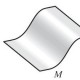Feeds:
Posts

## Kraft’s inequality for prefix codes

Kraft’s inequality: Let$L$ be a prefix code on an alphabet of size$k$, and let$l_n$ denote the number of codewords of length$n$. Then$\displaystyle \sum_{n \ge 1} \frac{l_n}{k^n} \le 1$.

In lieu of reposting my old blog posts I thought I’d revisit some of their topics instead. In an old post of mine I set this inequality as a practice problem. I had an easy but uninspired proof in mind, but recently I learned that this problem is an exercise in the first chapter of The probabilistic method by Alon and Spencer. The probabilistic proof is quite elegant, so I thought it was worth presenting.

The “follow-your-nose” proof

(Below “code” will mean “prefix code.”)

The idea behind the inequality is that there is a trade-off among codewords: having a particular small codeword forbids you from having many other larger codewords. Define the weight of a codeword of length$n$ to be$\frac{1}{k^n}$, and define the weight of a code to be the sum of the weights of its codewords. Then we want to show that prefix codes cannot be too heavy.

An example of a code of weight$1$ is the code$A$ consisting of precisely the letters of the alphabet. This code has the property that it is not possible to add more words to it without violating the prefix condition; call such a code maximal. Clearly any code is a subcode of a maximal code, so it suffices to show that every maximal code has weight at most$1$. (As we will see, they in fact have weight exactly$1$.)

Given a codeword of length$n$ one may replace it by all the codewords of length$n+1$ whose prefix is that codeword; call this lengthening the codeword. The important observation here is that lengthening a codeword leaves the weight of the code invariant; moreover, lengthening preserves the property of being a maximal code. The following claim will then prove the original assertion.

Claim: Any maximal code$C$ can be obtained from$A$ by a “convergent sequence” of lengthenings, in the sense that for any$n$ there exists$N$ such that at any time after the first$N$ lengthenings the codewords of length at most$n$ are correct.

Proof. This is an example of a just-do-it proof. Construct a sequence$A_1, A_2, ...$ of codes as follows: starting with$A_0 = A$, at step$n$ lengthen every codeword of length$n$ in$A_{n-1}$ that is not in$C$ and call the new code$A_n$. This algorithm guarantees that after step$n$ the codes$A_n$ agrees with$C$ at least up to codewords of length$n$, since lengthening a codeword of length$k$ can only cause “disagreements” for codewords of length at least$k+1$. And we are done.

The topology we’re using here is essentially the$t$-adic topology on the generating functions$\displaystyle \sum_{n \ge 0} l_n t^n$ of these codes. Consideration of these generating functions leads to another proof of this result, but the Wikipedia article does a good job of explaining it, so I won’t.

The probabilistic proof

Write down letters from the alphabet at random. If at any point you write down a codeword, stop. This defines a probability distribution on the set of codewords, and since no codeword is a prefix of another codeword the probability of reaching a codeword of length$n$ is exactly$\frac{1}{k^n}$.

It follows that$1 - \sum_{n \ge 1} \frac{l_n}{k^n}$ is the probability that this process never terminates.

Generalizations

The generating functions proof generalizes to the Kraft-McMillan inequality for uniquely decodable codes. This is essentially the reason people care about prefix codes: given a concatenation of codewords one can recover each codeword uniquely. This is the motivating idea behind the generating functions proof, but the Kraft-McMillan inequality is also listed as an exercise to be done via the probabilistic method. Unfortunately, I haven’t yet found this more general proof.

I am really curious whether the prefix-code result could be subsumed under a generalization of Dilworth’s theorem. A prefix code is an antichain in the poset of words ordered by prefix; what is the analogue of the size of a chain decomposition in this setting? Alternately, how can Dilworth’s theorem be stated (and proven?) as a probabilistic result?

### 5 Responses

1. on April 3, 2011 at 6:23 pm | ReplyAkhil Mathew

If you don’t care about generalizing to uniquely decodable codes, then Kraft’s inequality follows because a prefix code (say, a binary one) can be represented by a sequence of subintervals of $[0, 1]$. Namely, we map a sequence$a_0 \dots a_k$ to the interval of length$2^{-k}$ starting at$.a_0 \dots a_k$ (in the binary expansion). The prefix-free condition means that the intervals don’t overlap, so the fact their total measure is at most one is precisely the inequality in question.

2. […] known results, but clever applications can give upper bounds as well, for instance in the proof of Kraft’s inequality. Of particular interest to us is the fact that it provides our first nontrivial proof in the […]

3. on August 27, 2009 at 11:22 pm | ReplyCarnival of Mathematics #56 « Reasonable Deviations

[…] Yuan writes about Kraft’s inequality for prefix codes and the probabilistic method, and also on linear relationships between square […]

4. on August 12, 2009 at 6:41 pm | ReplyHarrison

Now that I think about it, the LYM inequality is another instance of this very general result that doesn’t actually seem to exist, and is way more obviously similar to the Kraft inequality.

5. on August 12, 2009 at 6:30 pm | ReplyHarrison

You probably thought of this already, but it should be stated outright: In particular, note that both Kraft’s inequality and Dilworth’s theorem have to do with maximal weights of antichains in a poset with weighted elements. (Dilworth’s theorem just sets all the weights to 1.)